• python数据分布拟合
2020-12-08 08:13:29

这是个复杂的问题，没有完美的答案，

对于给定的数据，有两种设置概率分布函数参数的方法：

在我的经验中，最近几年最大似然是首选的，尽管这可能不是每个领域的情况。

这是如何估算R中参数的具体示例。考虑从高斯分布生成的一组随机点，它均值为0，标准差为1：x = rnorm( n = 100, mean = 0, sd = 1 )

在R中，有一个标准库使这非常简单：library(MASS)

params = fitdistr( x,"normal" )

print( params )

这给了我以下输出：mean sd

-0.17922360 1.01636446

( 0.10163645) ( 0.07186782)

从你的参数中提取日志可能性，如下所示：print( params$loglik )  -139.5772 当对数可能性接近0时，可能性最大，因此负数越多，数据拟合效果越差。 使用这样的计算工具，可以很容易地估计分布的参数，请考虑以下示例：x = x[ x >= 0 ] distributions = c("normal","exponential") for ( dist in distributions ) { print( paste("fitting parameters for", dist ) ) params = fitdistr( x, dist ) print( params ) print( summary( params ) ) print( params$loglik )

}

指数分布不产生负数，所以我在第一行中删除了它们，输出(这是随机)如下所示："fitting parameters for normal"

mean sd

0.72021836 0.54079027

(0.07647929) (0.05407903)

Length Class Mode

estimate 2 -none- numeric

sd 2 -none- numeric

n 1 -none- numeric

loglik 1 -none- numeric

 -40.21074

"fitting parameters for exponential"

rate

1.388468

(0.196359)

Length Class Mode

estimate 1 -none- numeric

sd 1 -none- numeric

n 1 -none- numeric

loglik 1 -none- numeric

 -33.58996

克服参数估计中一些问题的一个技巧是生成大量数据，并将某些数据留给交叉验证。

更多相关内容
• 安装fitter pip install fitter 生成一段模拟数据 from scipy import stats import numpy as np # N(0,2)+N(0,10) data1 = list(stats.norm.rvs(loc=0, scale=2, size=70000)) ...利用fitter拟合数据

## 安装fitter

pip install fitter

## 生成一段模拟数据

from scipy import stats
import numpy as np
# N(0,2)+N(0,10)
data1 = list(stats.norm.rvs(loc=0, scale=2, size=70000))
data2 = list(stats.norm.rvs(loc=0, scale=20, size=30000))
data=np.array(data1+data2)


## 利用fitter拟合数据样本的分布

from fitter import Fitter
# may take some time since by default, all distributions are tried
# but you call manually provide a smaller set of distributions
f = Fitter(data, distributions=['norm', 't', 'laplace'])
f.fit()
f.summary()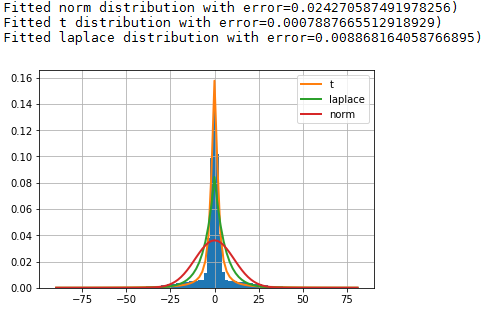以上输出为拟合的误差与拟合的概率密度曲线及数据的直方图。

## 方法详解

### Fitter方法

Fitter(data, xmin=None, xmax=None, bins=100, distributions=None, verbose=True, timeout=10)


参数：
data (list) –输入的样本数据；
xmin (float) – 如果为None，则使用数据最小值，否则将忽略小于xmin的数据；
xmax (float) – 如果为None，则使用数据最大值，否则将忽略大于xmin的数据；
bins (int) – 累积直方图的组数，默认=100；
distributions (list) – 给出要查看的分布列表。 如果没有，则尝试所有的scipy分布(80种),常用的分布distributions=[‘norm’,‘t’,‘laplace’,‘cauchy’, ‘chi2’,’ expon’, ‘exponpow’, ‘gamma’,’ lognorm’, ‘uniform’]；
verbose (bool) –
timeout – 给定拟合分布的最长时间，（默认=10s） 如果达到超时，则跳过该分布。

### Fitter返回

f.summary() #返回排序好的分布拟合质量（拟合效果从好到坏）,并绘制数据分布和Nbest分布
f.df_errors #返回这些分布的拟合质量（均方根误差的和）
f.fitted_param #返回拟合分布的参数
f.fitted_pdf #使用最适合数据分布的分布参数生成的概率密度
f.get_best(method='sumsquare_error') #返回最佳拟合分布及其参数
f.hist() #绘制组数=bins的标准化直方图
f.plot_pdf(names=None, Nbest=3, lw=2) #绘制分布的概率密度函数

from fitter import Fitter
import numpy as np

arr = np.arange(1, 200)
np.random.shuffle(arr) # arr为创建的随机数

fitter_dis = Fitter(arr)
fitter_dis.fit()
distribution_df = fitter_dis.summary() # 这里可以得到error最小的Dataframe型数据

展开全文• Python fitter包：拟合数据样本的分布安装fitterFitter方法参数详解HistFit类：适合密度函数本身Python拟合数据样本的分布 github项目：https://github.com/cokelaer/fitter fittle 说明文档： ...

### Python fitter包：拟合数据样本的分布

github项目：https://github.com/cokelaer/fitter

### 安装fitter

pip install fitter


生成一段模拟数据

from scipy import stats
data = stats.gamma.rvs(2, loc=1.5, scale=2, size=100000)


利用fitter拟合数据样本的分布

from fitter import Fitter
# may take some time since by default, all distributions are tried
# but you call manually provide a smaller set of distributions
f = Fitter(data, distributions=['gamma', 'rayleigh', 'uniform'])
f.fit()
f.summary()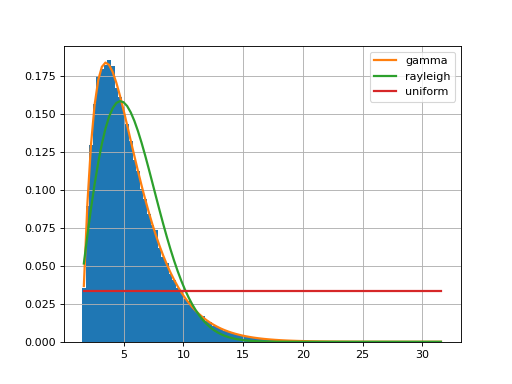以上输出为拟合的误差与拟合的概率密度曲线及数据的直方图。

### Fitter方法参数详解

Fitter(data, xmin=None, xmax=None, bins=100, distributions=None, verbose=True, timeout=10)


参数：
data (list) –输入的样本数据；
xmin (float) – 如果为None，则使用数据最小值，否则将忽略小于xmin的数据；
xmax (float) – 如果为None，则使用数据最大值，否则将忽略大于xmin的数据；
bins (int) – 累积直方图的组数，默认=100；
distributions (list) – 给出要查看的分布列表。 如果没有，则尝试所有的scipy分布(80种),常用的分布distributions=[‘norm’,‘t’,‘laplace’,‘cauchy’, ‘chi2’,’ expon’, ‘exponpow’, ‘gamma’,’ lognorm’, ‘uniform’]；
verbose (bool) –
timeout – 给定拟合分布的最长时间，（默认=10s） 如果达到超时，则跳过该分布。
Fitter返回

f.summary() #返回排序好的分布拟合质量（拟合效果从好到坏）,并绘制数据分布和Nbest分布
f.df_errors #返回这些分布的拟合质量（均方根误差的和）
f.fitted_param #返回拟合分布的参数
f.fitted_pdf #使用最适合数据分布的分布参数生成的概率密度
f.get_best(method='sumsquare_error') #返回最佳拟合分布及其参数
f.hist() #绘制组数=bins的标准化直方图
f.plot_pdf(names=None, Nbest=3, lw=2) #绘制分布的概率密度函数


一旦执行了拟合，就可能想要获得与最佳分布相对应的参数。参数存储在中fitted_param。例如，在上面的示例中，摘要告诉我们Gamma分布最适合。您将按以下方式检索Gamma分布的参数：

>>> f.fitted_param['gamma']
(1.9870244799532322, 1.5026555566189543, 2.0174462493492964)


在这里，您将需要查看scipy文档以找出那些参数（均值，sigma，shape等）。为了方便起见，我们提供相应的PDF：

f.fitted_pdf['gamma']


但您可能想自己绘制伽马分布。在这种情况下，您将需要使用Scipy软件包本身。这是一个例子

from pylab import linspace, plot
import scipy.stats

dist = scipy.stats.gamma
param = (1.9870, 1.5026, 2.0174)
X = linspace(0,10, 10)
pdf_fitted = dist.pdf(X, *param)
plot(X, pdf_fitted, 'o-')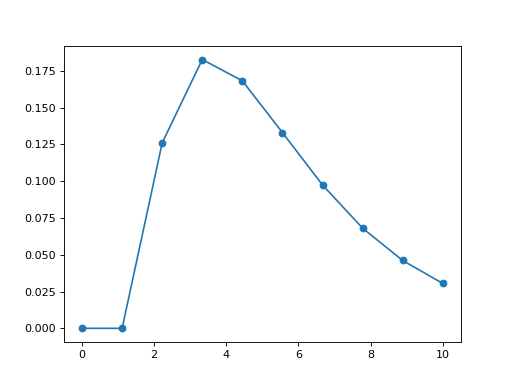https://www.freesion.com/article/9309375330/

### HistFit类：适合密度函数本身

有时，您只有发行版本身。例如：

import scipy.stats
data = [scipy.stats.norm.rvs(2,3.4) for x in  range(10000)]
Y, X, _ = hist(data, bins=30)


在这里，我们只能访问Y（和X）。

histfit模块提供了HistFit类，可基于多次尝试对X / Y数据进行拟合而在数据集上存在一些错误，从而使用拟合曲线生成数据图。例如，在下面的示例中，我们引入3％的错误，并拟合20次数据以查看拟合是否有意义。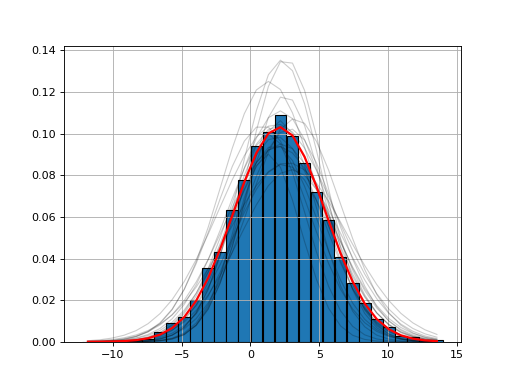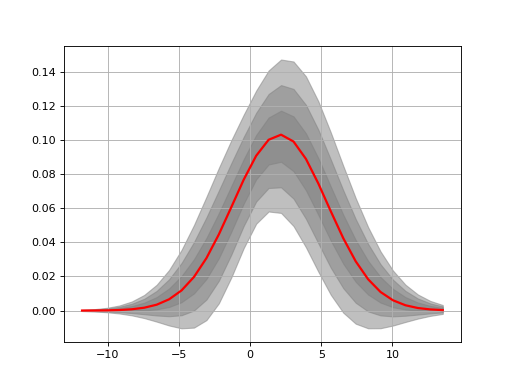### Python拟合数据样本的分布

# Python拟合数据样本的分布
# 安装fitter
# pip install fitter
# 生成一段模拟数据
from scipy import stats
import numpy as np
# N(0,2)+N(0,10)
data1 = list(stats.norm.rvs(loc=0, scale=2, size=70000))
data2 = list(stats.norm.rvs(loc=0, scale=20, size=30000))
data = np.array(data1+data2)

# 利用fitter拟合数据样本的分布
from fitter import Fitter
# may take some time since by default, all distributions are tried
# but you call manually provide a smaller set of distributions
f = Fitter(data, distributions=['norm', 't', 'laplace', 'rayleigh'])
f.fit()
f.summary() #返回排序好的分布拟合质量（拟合效果从好到坏）,并绘制数据分布和Nbest分布
f.df_errors #返回这些分布的拟合质量（均方根误差的和）
f.fitted_param #返回拟合分布的参数
f.fitted_pdf #使用最适合数据分布的分布参数生成的概率密度
print(f.get_best(method='sumsquare_error')) #返回最佳拟合分布及其参数
f.hist() #绘制组数=bins的标准化直方图
f.plot_pdf(names=None, Nbest=3, lw=2) #绘制分布的概率密度函数
print(f.summary())

# 方法详解
# Fitter方法
# Fitter(data, xmin=None, xmax=None, bins=100, distributions=None, verbose=True, timeout=10)
# 参数：
# data (list) –输入的样本数据；
# xmin (float) – 如果为None，则使用数据最小值，否则将忽略小于xmin的数据；
# xmax (float) – 如果为None，则使用数据最大值，否则将忽略大于xmin的数据；
# bins (int) – 累积直方图的组数，默认=100；
# distributions (list) – 给出要查看的分布列表。 如果没有，则尝试所有的scipy分布(80种),常用的分布distributions=[‘norm’,‘t’,‘laplace’,‘cauchy’, ‘chi2’,’ expon’, ‘exponpow’, ‘gamma’,’ lognorm’, ‘uniform’]；
# verbose (bool) –
# timeout – 给定拟合分布的最长时间，（默认=10s） 如果达到超时，则跳过该分布。

# Fitter返回
# f.summary() #返回排序好的分布拟合质量（拟合效果从好到坏）,并绘制数据分布和Nbest分布
# f.df_errors #返回这些分布的拟合质量（均方根误差的和）
# f.fitted_param #返回拟合分布的参数
# f.fitted_pdf #使用最适合数据分布的分布参数生成的概率密度
# f.get_best(method='sumsquare_error') #返回最佳拟合分布及其参数
# f.hist() #绘制组数=bins的标准化直方图
# f.plot_pdf(names=None, Nbest=3, lw=2) #绘制分布的概率密度函数

# from fitter import Fitter
# import numpy as np
#
# arr = np.arange(1, 200)
# np.random.shuffle(arr)  # arr为创建的随机数
#
# fitter_dis = Fitter(arr)
# fitter_dis.fit()
# distribution_df = fitter_dis.summary()  # 这里可以得到error最小的Dataframe型数据

展开全文python
• 我希望使用Python 3.4为一组数据找到最合适的weibull参数.import scipy.stats as sslist1 = []list2 = []for x in range(0, 10):list1.append(ss.exponweib.pdf(x, a=1, c=2.09, scale=10.895, loc=0))list2.append...

我希望使用Python 3.4为一组数据找到最合适的weibull参数.

import scipy.stats as ss

list1 = []

list2 = []

for x in range(0, 10):

list1.append(ss.exponweib.pdf(x, a=1, c=2.09, scale=10.895, loc=0))

list2.append(ss.weibull_min.pdf(x, c=2.09, loc=0, scale=10.895))

if list1[x]-list2[x] < .000000001:

list1[x]=list2[x]

if list1 == list2:

print("true")

print(ss.distributions.weibull_min.fit(list1, floc=0))

print(ss.distributions.weibull_min.fit(list1, loc=0))

print(ss.distributions.weibull_min.fit(list1, floc=0))

print(ss.distributions.exponweib.fit(list1, 1,1))

print(ss.distributions.exponweib.fit(list1, floc=0, f0=1))

print(ss.distributions.exponweib.fit(list1, floc=0, a=1, f0=1))

我尝试过的所有内容都没有产生输入参数,我无法弄清楚原因.

此代码的输出是：

true

(2.8971366871403661, 0, 0.065615284314998634)

(0.71134622938358294, 0.014105558832066645, 0.076662586739229072)

(2.8971366871403661, 0, 0.065615284314998634)

(0.27753056922336583, 3.1962672780921197, -3.4788071110631162e-27, 0.077986010645321888)

(1, 2.8971366871403661, 0, 0.065615284314998634)

(1, 2.8971366871403661, 0, 0.065615284314998634)

这些都不是正确的输入参数. (2.09和10.895.)任何帮助表示赞赏.谢谢.

解决方法:

fit()方法的第一个参数是要适合的分布的值的样本(不是PDF值).所以你应该使用rvs()方法来生成数据,而不是pdf()方法.

这是一个简单的例子,我从exponweib发行版中生成250个值的样本,然后在该样本上使用fit().我假设当我拟合数据时,我知道形状参数a必须为1且loc参数必须为0：

In : from scipy.stats import exponweib

In : sample = exponweib.rvs(a=1, c=2.09, scale=10.895, loc=0, size=250)

In : exponweib.fit(sample, floc=0, fa=1)

Out: (1, 2.0822583185068915, 0, 10.946962241403902)

标签：python,python-3-x,scipy

展开全文• ## Python数据正态拟合

千次阅读 2019-12-13 16:02:53
from scipy.stats import norm#使用直方图和最大似然高斯分布拟合绘制分布 #rs=np.random.RandomState(50)#设置随机数种子 #s=pd.Series(rs.randn(100)*100) s=np.loadtxt('D:/dis.txt') mu =np.mean(s) #计算均值 ...
• 所以，判定数据是否符合幂律分布，只需要对XY取双对数，判断能否用一个直线很好拟合就行。常见的直线拟合效果评估标准有拟合误差平方和、R平方。 3、代码实现 #!/usr/bin/env python # -*-coding:utf-8 -*- import ...
• 使用Python进行数据拟合 文章目录使用Python进行数据拟合多项式拟合非多项式拟合 多项式拟合 任何一个函数都可以拆分成近似于这个函数的多项式表达。 多项式拟合需要用到的函数是np.polyfit，它的使用方法为： np...python
• 先写一个摇色子的函数 from random import randint class die: def __init__(self,num_sides = 6): self.num_sides = num_sides def roll(self): return randint(1... 运行结果： 随着次数增加，不断逼近正态分布函数。
• 对来自总体XXX的样本X1,X2,⋯ ,XnX_1,X_2,\cdots,X_nX1​,X2​,⋯,Xn​，及给定的显著水平α\alphaα检验假设H0:X的分布函数为F(x)(H1:X的分布函数不是F(x)).H_0:X\text{的分布函数为}F(x)(H_1:X\text{的分布函数...
• 直方图是用于展示数据的分组分布状态的一种图形，用矩形的宽度和高度表示频数分布，通过直方图，用户可以很直观的看出数据分布的形状、中心位置以及数据的离散程度等。 在python中一般采用matplotlib库的hist来绘制...
• 小李默默吐槽，被这个高斯拟合分布折磨的吐血，下面是正文 首先是公式 # 自定义函数 def func(x, a, u, sig): return a*(np.exp(-(x - u) ** 2 / (2 * sig ** 2))) 然后就是横纵坐标，画图 x = np.array(dist) ...python
• ## 概率分布拟合

千次阅读 2021-02-04 04:41:27
我试图让数据符合概率分布(在我的例子中是伽马函数)。在用瞬间法我取得了一些成功：mean, var = data.mean(), data.var()α, β = mean ** 2 / var, var / meanx = np.linspace(0, 100)plt.plot(x, gamma.pdf(x, α,...
• 一、多项式拟合 多项式拟合的话，用的的是numpy这个库的polyfit这个函数。那么多项式拟合，最简单的当然是，一次多项式拟合了，就是线性回归。直接看代码吧 import numpy as np def linear_regression(x,y): #y=bx...
• 主要介绍了python 对任意数据和曲线进行拟合并求出函数表达式的三种解决方案,本文通过实例代码给大家介绍的非常详细，具有一定的参考借鉴价值，需要的朋友可以参考下
• 正态分布（Normal distribution）又成为高斯分布（Gaussian distribution） ...# Python实现正态分布 # 绘制正态分布概率密度函数 u = 0 # 均值μ u01 = -2 sig = math.sqrt(0.2) # 标准差δ sig01 = mat
• 如何用python画出直方图的包络线拟合直方图与Python问题，怎么解决用代码解决： import numpy as np import matplotlib.pyplot as plt from scipy.interpolate import interp1d import scipy.stats as st sim = st....
• 1.安装 pip(3) install fitter 2.例子 # 数据生成 from scipy import stats ...# 拟合分布 from fitter import Fitter f = Fitter(data) # 创建Fitter类 f.fit() # 调用fit函数拟合分布 f.summary() # 输出拟合python 开发语言 后端
• ## Python scipy拟合分布

千次阅读 2021-03-29 14:24:02
方差为5的正态分布数据 4000个 dist = getattr(stats, 'norm') parameters = dist.fit(number) # 或者使用 # parameters = stats.norm.fit(number) print(parameters) # 输出(9.991796870780716, 4....scipy
• 相比numpy库的多项式拟合函数polyfit，scipy.optimize模块中的函数curve_fit能够拟合的函数fang'we，不局限于多项式函数python scipy
• python拟合对数正态分布使用的是scipy.stats.lognorm这个包，这个包的使用看官方文档就行，但是其中有一个很迷的地方，网上也有人提到了这个很迷的地方：关于scipy对数正态分布的误区，然后Stack Overflow里也有人...python scipy 对数正态分布
• 我试图用statsmodels将Poisson分布拟合到我的数据中，但是我对得到的结果和如何使用库感到困惑。我的真实数据将是一系列的数字，我认为我应该能够描述为泊松分布加上一些离群值，所以最终我想做一个稳健的数据拟合。...
• 韦伯分布的定义拟合方法的原理python代码实现参考python
• 我试图使用Python获得数据的双高斯分布(link).原始数据的格式如下： 对于给定的数据,我想获得图中所示峰值的两个高斯分布.我尝试使用以下代码(source)：from sklearn import mixtureimport matplotlib.pyplotimport ...
• 因为curvefit给你一个常量(一条直线)，因为你给它传递的是一个使用你定义的模型不相关的数据集！在让我先重新创建您的设置：argon = np.genfromtxt('argon.dat')copper = np.genfromtxt('copper.dat')f1 = 1 - np....
• 第二条曲线用了正态分布函数曲线进行拟合。 import numpy as np import pandas as pd import matplotlib.pyplot as plt datas = np.array([64.3, 65.0, 65.0, 67.2, 67.3, 67.3, 67.3, 67.3, 68.0, 68.0, 68.8, ...python
• # Fitter返回 # f.summary() #返回排序好的分布拟合质量（拟合效果从好到坏）,并绘制数据分布和Nbest分布 # f.df_errors #返回这些分布的拟合质量（均方根误差的和） # f.fitted_param #返回拟合分布的参数 # f....python
• How can I make such a test in Python? 解决方案 The Lilliefors test is implemented in OpenTURNS. To do this, all you have to use the Factory which corresponds to the distribution you want to fit. In ...
• ## Python Numpy泊松分布

千次阅读 2020-12-21 19:30:41
首先，假设您import numpy as np，我会写下这个答案，因为它明确区分numpy函数与内置函数或math和random的函数包的python。我认为没有必要回答您指定的问题，因为您的基本假设是错误的：是的，泊松统计量的均值等于......